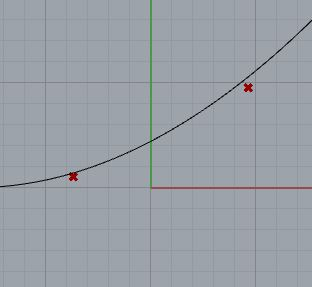# Apparent Intersection of curve and line

Hi All,

Long time programmer in AutoCAD, first time programmer and poster in GH forums.

I am trying to create a component where I have a Curve (Non-Planar) and I am trying to find the intersection point of the two (I want to get the X & Y of where the curve intersects the line, but get Z value of the curve at that point).

Currently, I am trying to project the curve to Z = 0, by iterating it
double increment = 0.5;
double oaLength = 0;
List points = new List();
do
{
Point3d newpt = controlString.PointAtLength(oaLength);
oaLength = oaLength + increment;
}
while (oaLength < controlString.GetLength());
Curve flattenedCurve = Curve.CreateControlPointCurve(points);

then finding the intersection point on the flattened curve
var events = Intersection.CurveLine(flattenedCurve, line, intersectionTolerance, overlapTolerance);

``````        if (events != null)
{
if (events.Count > 0)
{
var ccx_event = events;
pointOnFlattenedCurve = ccx_event.PointA;
}
}
``````

then going along the original curve to find a point
double increment = 0.5;
double oaLength = 0;

``````        do
{
Point3d testPointOnControlString = controlString.PointAtLength(oaLength);
Point3d testPointAtZeroElevation = new Point3d(testPointOnControlString.X, testPointOnControlString.Y, 0);
double dist = pointOnFlattenedCurve.DistanceTo(testPointAtZeroElevation);
if (dist < 1)
{
return new Point3d(pointOnFlattenedCurve.X, pointOnFlattenedCurve.Y, testPointOnControlString.Z); ;
}
oaLength = oaLength + increment;
}
while (oaLength < controlString.GetLength());

return controlString.PointAtStart;
``````

The points are not exactly lining up on the curve (on the Z-Axis).

Is there an easier way to get this intersection point?

Intersection Point.3dm (23.4 KB)``````var pl = new Plane(l.To, l.Direction, v);
var intersections = Intersection.CurvePlane(c, pl, Rhino.RhinoDoc.ActiveDoc.ModelAbsoluteTolerance);
var pts = new List<Point3d>();
foreach(var intersection in intersections)
if(intersection.IsPoint)